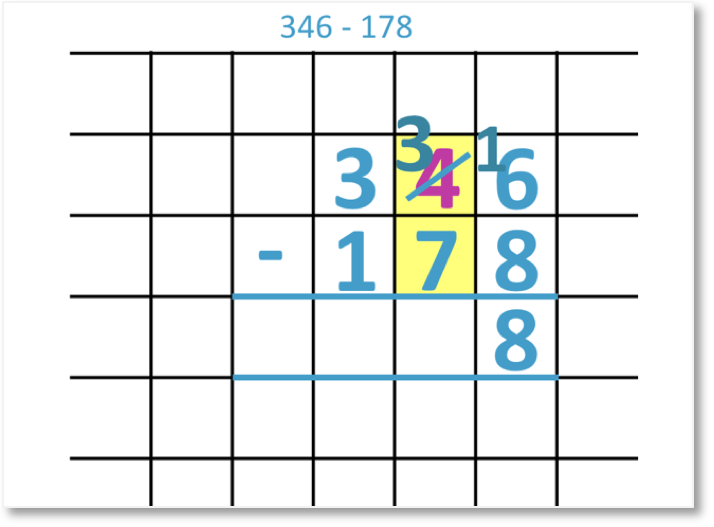# Column Subtraction of Three-Digit Numbers with Borrowing Twice

Column Subtraction: Video Lesson – Maths with Mum

Supporting Lessons

## How to do column subtraction when we borrow twice?

We will look at how to calculate 346 – 178.We begin by lining up the digits of each number in their place value columns. We start with the units column.

We want to subtract 8 from 6. However, 8 is greater than 6, so we can’t take it away.We must therefore borrow some units from the tens column. We can borrow from the 4 tens.We move 1 ten next to the 6. We have 16 units in total. We are left with 3 tens in the tens column.We can now subtract the units.

16 – 8 = 8

Next, we look at the tens column.We want to subtract the 7 from the 3. However, 7 is greater than 3, so we can’t.We must therefore borrow from the hundreds column. We can borrow from the 3 hundreds.We move 1 hundred next to the 3 in the tens column. This makes 13 in the tens column. We are left with 2 hundreds in the hundreds column.We can now subtract the tens.

13 – 7 = 6

Finally, we look at the hundreds column.Because we borrowed 1 hundred, we are left with 2 hundreds.

2 – 1 = 1

Therefore,

346 – 178 = 168.error: Content is protected !!